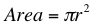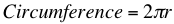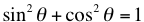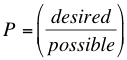# Math Formulas to Know by Heart

09/21/2015

## Math Help: Learn these Math Formulas

As you move ahead in your math career, you will find certain operations and problems continue to pop up. Here are some formulas you should memorize to help you advance quickly.

### Circle FormulasThe area of a circle is the square of the radius times π.Multiply π times the diameter, or twice the radius, of a circle. When working with arcs, just remember that an arc is a percentage of a circle. Since there are 360 degrees in a circle, first calculate the percentage of the whole circle the arc represents. If the arc is 90 degrees, it is 90/360=1/4 or 25%. Multiply the area or circumference of the whole circle by this percentage to find the area or circumference of the arc.

### SOHCAHTOA

To find the sine, cosine, and tangent of an angle, remember SOHCAHTOA. Sine= Opposite/Hypotenuse Cosine= Adjacent/Hypotenuse Tangent=Opposite/Adjacent Opposite is the side of the triangle directly opposite the angle in question. Adjacent is the side of the triangle next to the angle that is not the hypotenuse. The hypotenuse is the longest side of the triangle. It is also useful to know the reciprocals. Cosecant is the reciprocal of sine= Hypotenuse/Opposite Secant is the reciprocal of cosine= Hypotenuse/Adjacent Cotangent is the reciprocal of tangent= Adjacent/Opposite And finally, the square of an angles sine plus the square of an angle's cosine is 1 and the tangent is the quotient of the sine and cosine.### ProbabilityThe probability (P) of an event occurring is measured as a fraction (between 0 and 1) with 1 being 100% probability and 0 being 0%. Take the number of desired outcomes and divide by the number of possible outcomes. For example, if you want to know the probability of rolling a 3 on a six-sided di, it is 1 (because there is only one 3) over 6 (because there are 6 possibilities). To find the probability two, mutually exclusive events both occurring, multiply their probabilities. If you want to know the probability of rolling a 3 and then a 4, you would multiply 1/6*1/6 for 1/36.

## For Tutoring in Franklin Park

When you need additional help with math, contact The Tutoring Center in Franklin Park. We offer individualized one-to-one tutoring help in math, reading, writing, test prep, and more. Visit our webpage and call 732 419 3813 to schedule a free diagnostic assessment.

### FREE CONSULTATION

Schedule your Free Diagnostic Assessment Today!